# Python Variables

Variables in Python can reference values of different data types such as strings, integers, floating point values, Booleans, and different data types and data structures. Python, in contrast to statically typed languages such as Java or C++, doesn't require you to pre-declare a variable's data type. It determines the type during runtime.

### Values

Python supports several different types of values. These values are what variables can be assigned to.

Step 1 : Numeric Values – Integers

``````4 + 6 + 2 - 3
6*4``````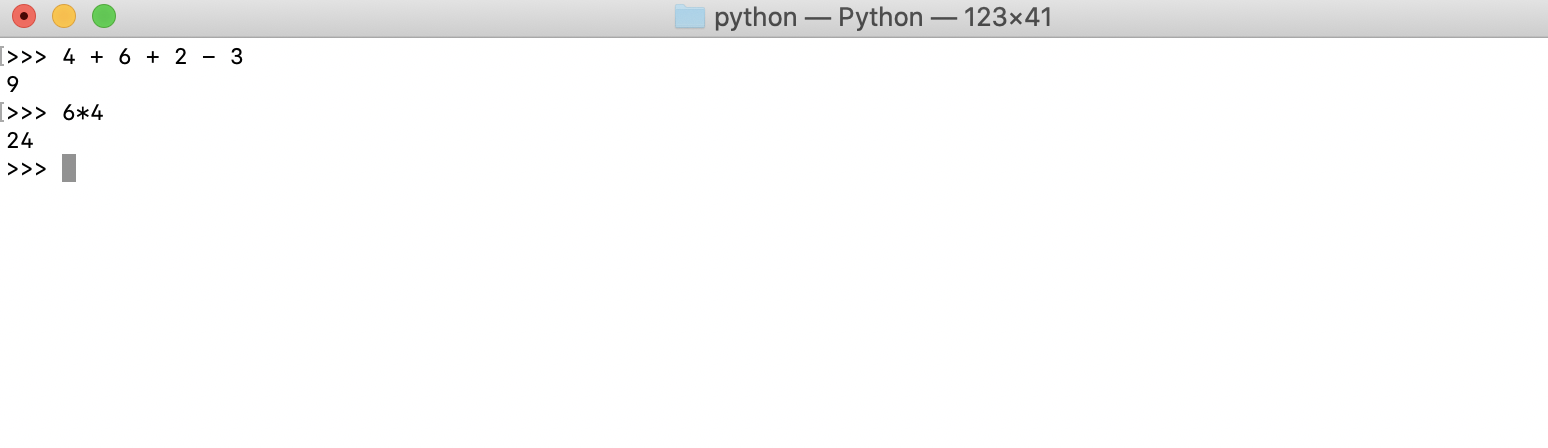Step 2 : String Values

``````type("iphone")## Checking the Type of a Value

Step 1 : We can also check the type of a value by using the type function that's built into Python. Enter the following code to view the type of the numeric value 23.

``type(23)``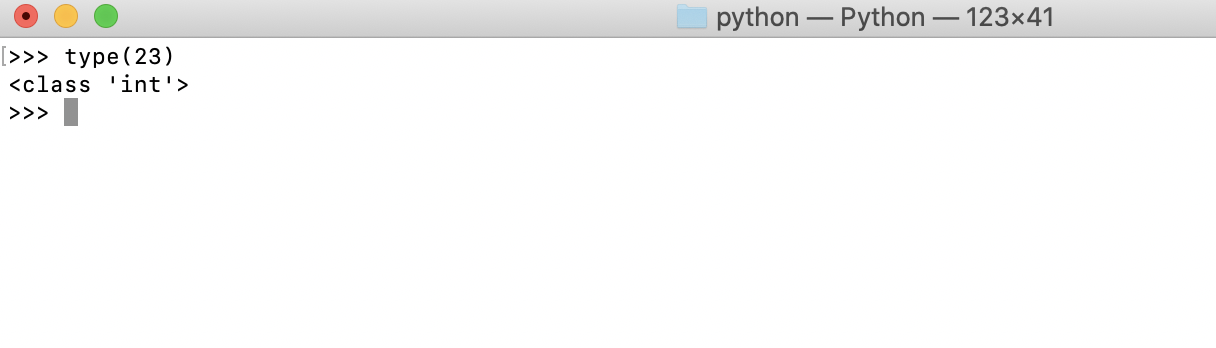Step 2 : Now, enter the following code, and observe the output

``type(41+4)``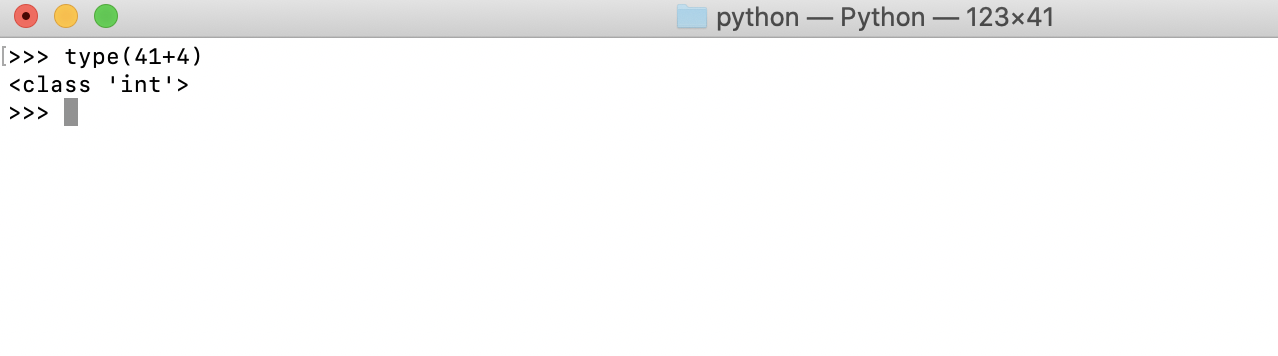Step 3 : Enter the following code at the prompt, and observe the output:

``````type("23")
type('23')``````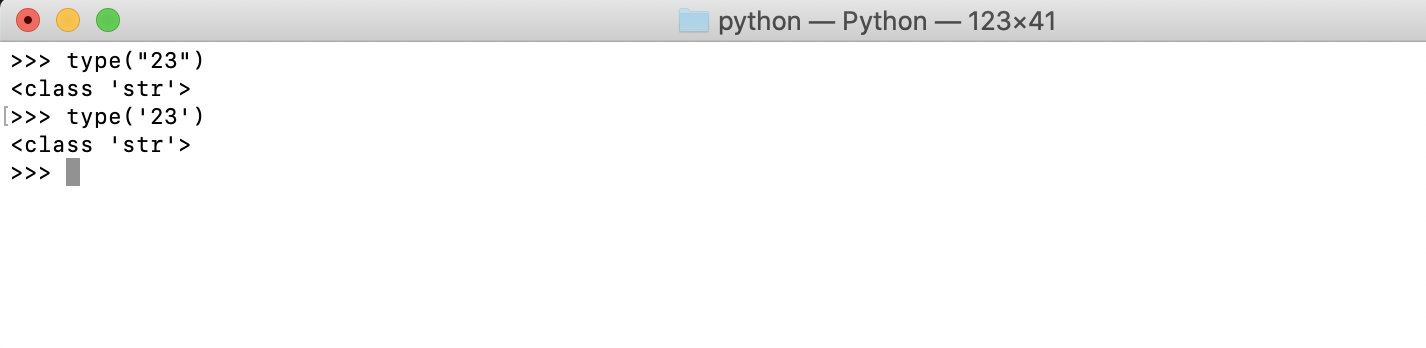## Type Conversion

Step 1 : Python allows you to convert string type values to integer type values and vice versa. Using the built-in str function, you can convert an integer to a string:

``str(23)``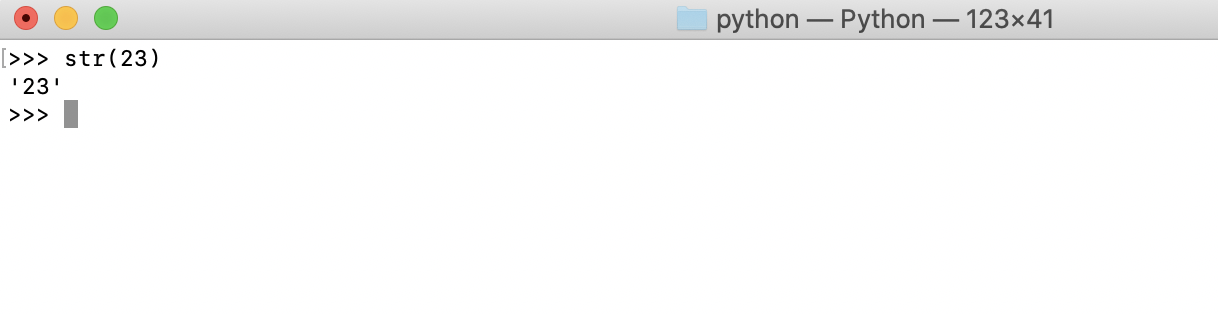Step 2 : Strings can also be converted to integers, as long as they hold a valid integer value within. This is done by use of the built-in int function:

``int("140")``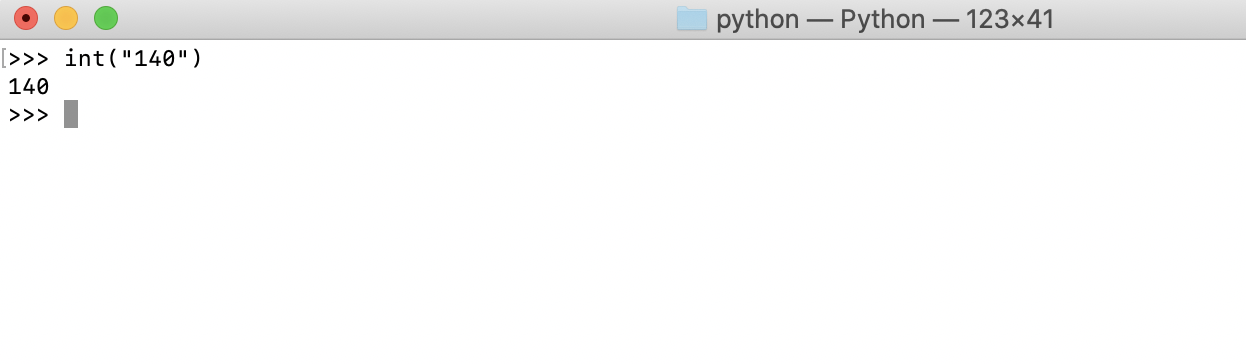Step 3 : An error occurs if we try converting a string that doesn't contain an integer.

``````int("Iphone")
int("7.6")``````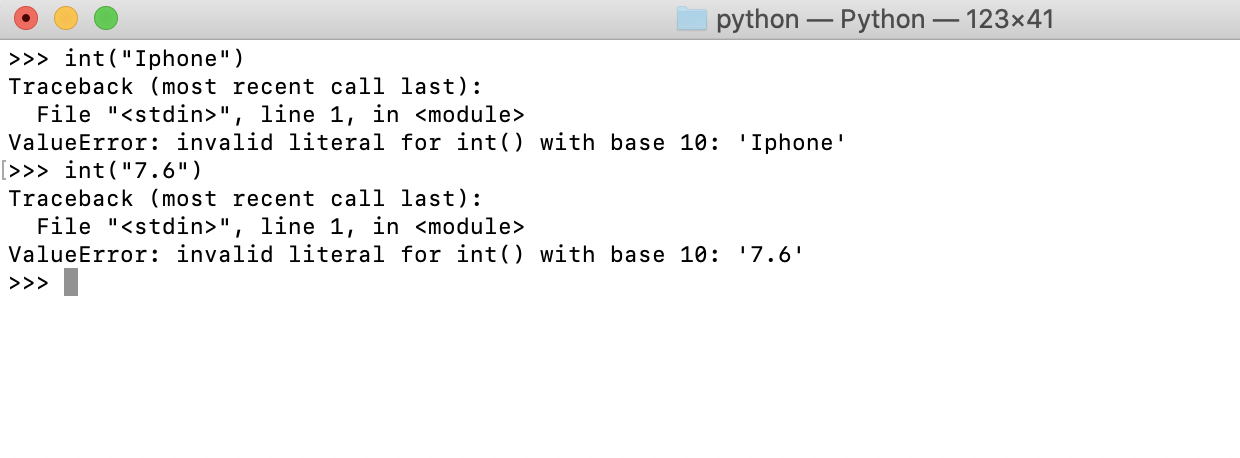## Assigning Variables

Step 1 : Assign a value to a variable in Python using the following synta

``number = 23``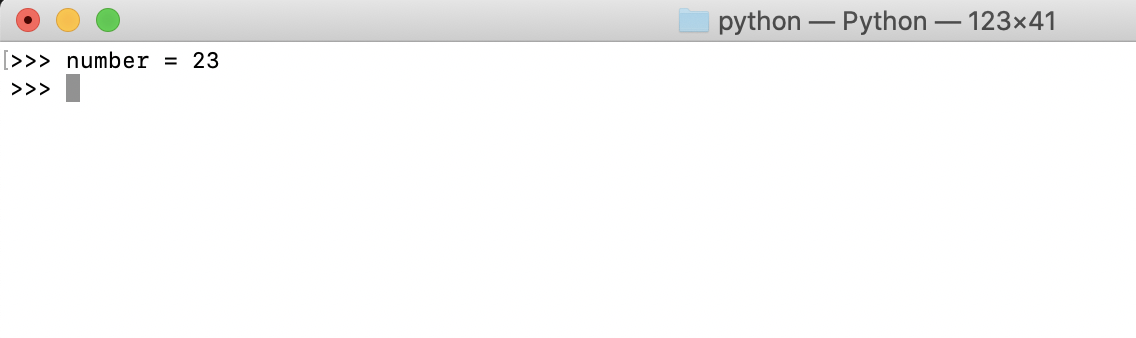Step 2 : Print the variable to the standard output; this should reveal its value

``print(number)``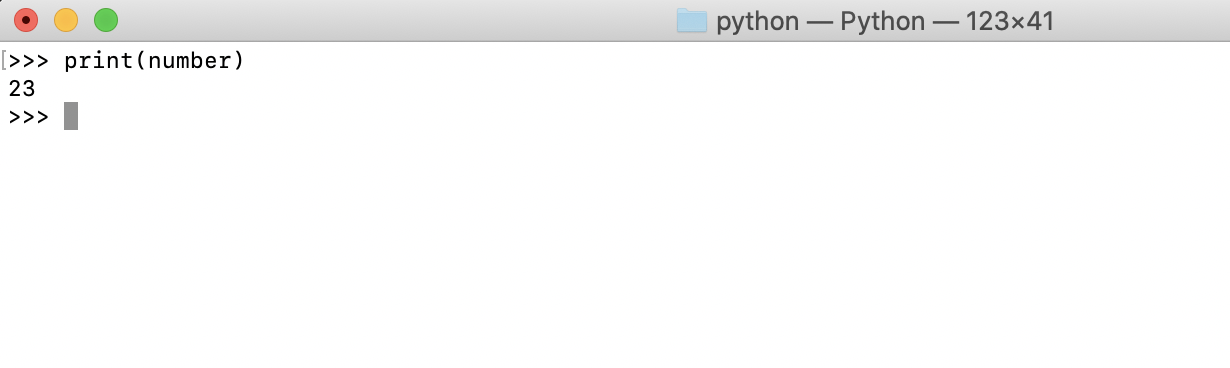Step 3 : However, if we try using a variable before assigning it a value, the Python interpreter will raise an error. Check this as follows

``````del number
print(number)``````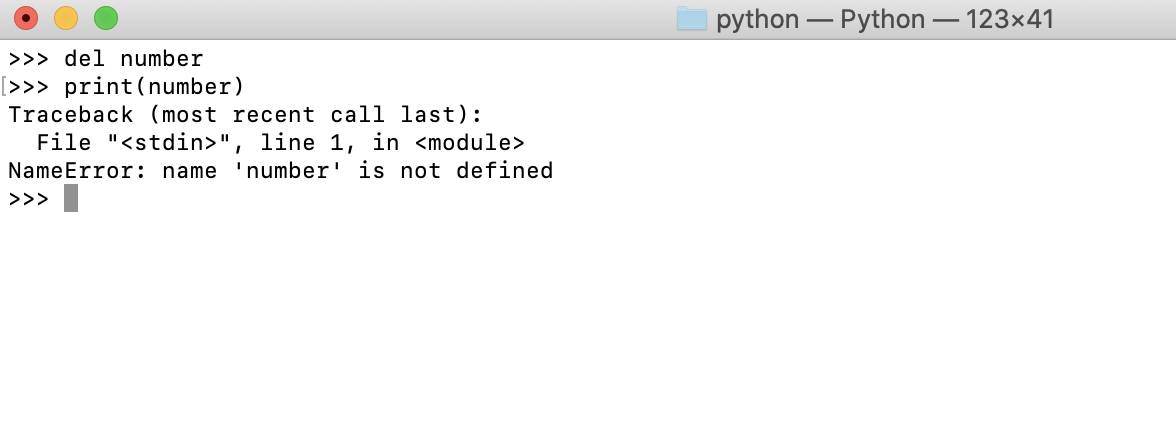## Using Variables

We use variables when we have a value in our code that we want to use multiple times. They prevent us from having to repeat that value each time we want to use it, as variables store the values in memory.

Step 1 : Assign the value 23 to the number variable

``number = 23``

Step 2 : We can now use this variable for any operations we'd like. Print out the value of the number variable, multiplied by 8

``number * 8``

Step 3 : Print out number added to 4

``number + 4``

Step 4 : Print out number divided by 2.5

``number / 2.5``

Step 5 : Print out number subtracted from itself

``number - number``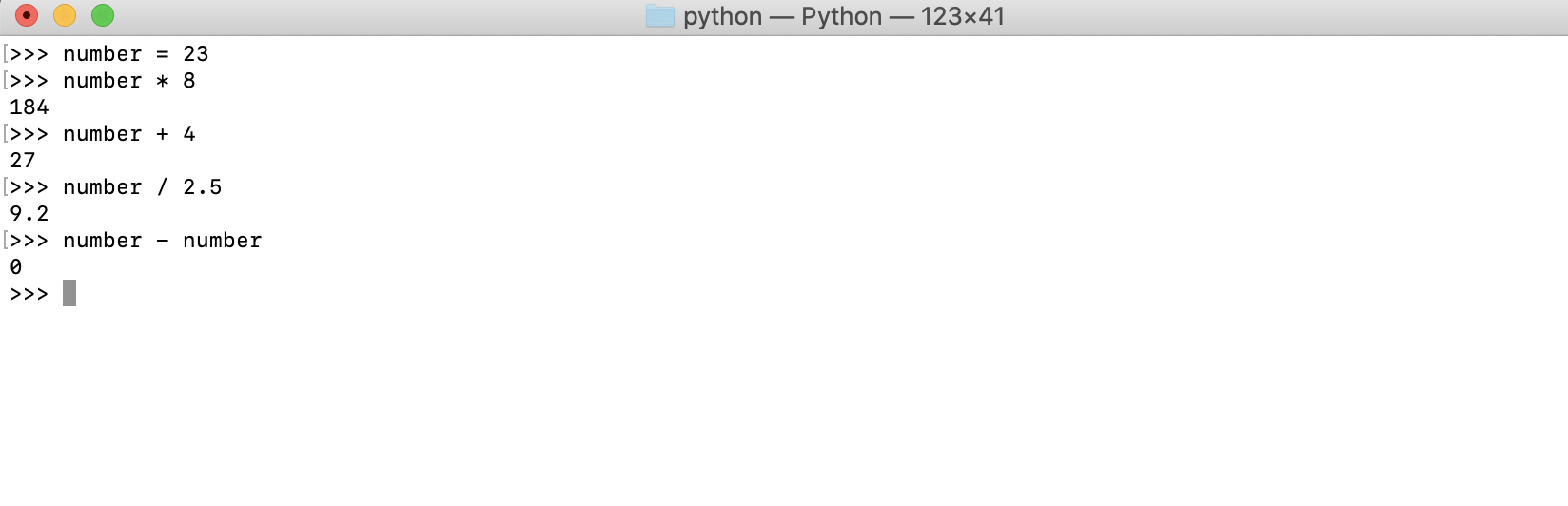Step 6 : Note that, despite having used it, the value of number won't change unless we reassign it. Reassigning 57 to number changes its value and verifies this:

``````print(number)
number = 57
print(number)``````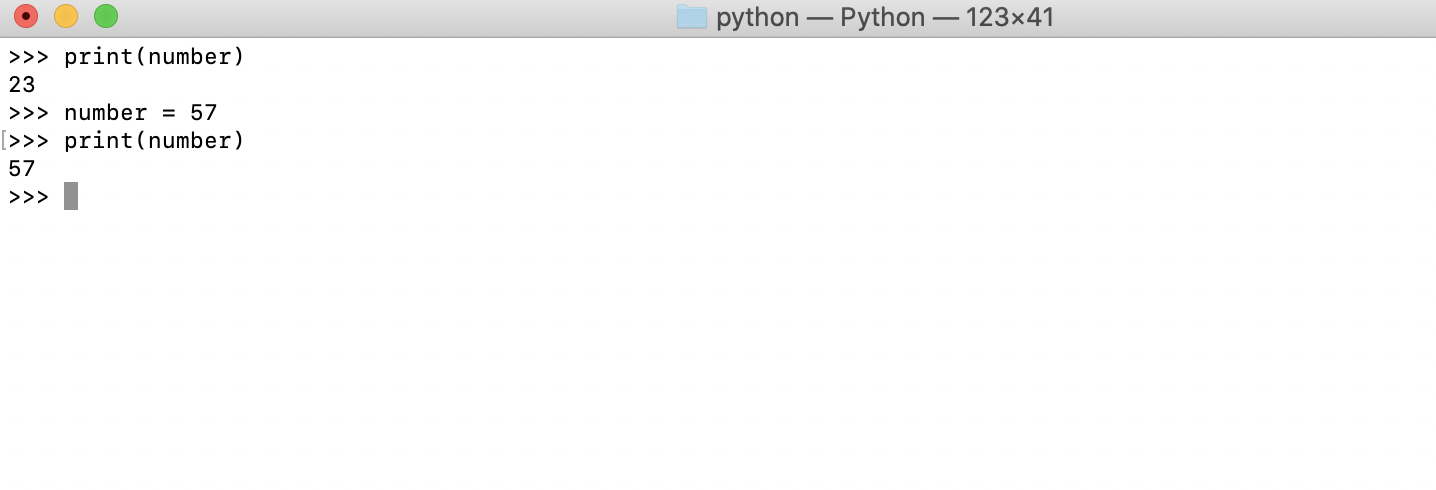Step 7 : You can also assign the resulting value of another operation to a variable

``````number = 24
x = number + 2
x``````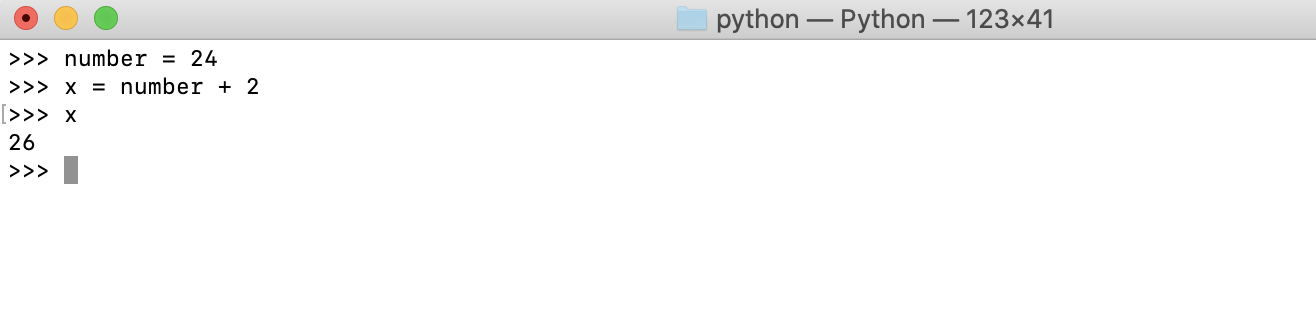Step 8 : String values can also be assigned and used in a similar fashion

``message = "iPhone"``

Step 9 : Print out the value of the message variable and add an exclamation point at the end

``message + "!"``

Step 10 : Print out message plus three exclamation points:

``message + "!" * 3``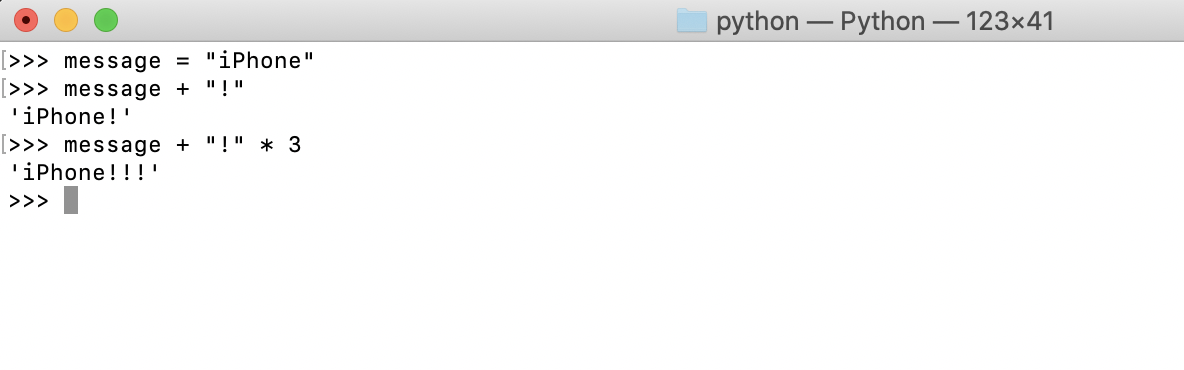## Multiple Assignment

Step 1 : In Python, you can also assign multiple variables in one statement, like so

``````a, b, c = 1, 2, 3
print(a)
print(b)
print(c)``````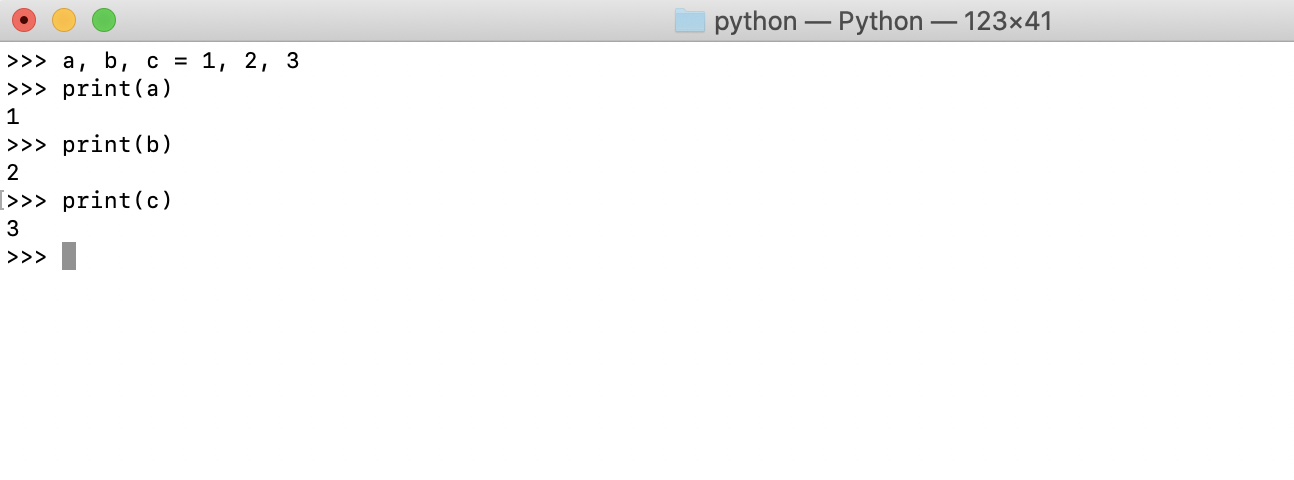Step 2 : The Python interpreter raises an error and tells us it didn't get enough values to assign to the variables we declared in our statement

``a, b = 1, 2, 3``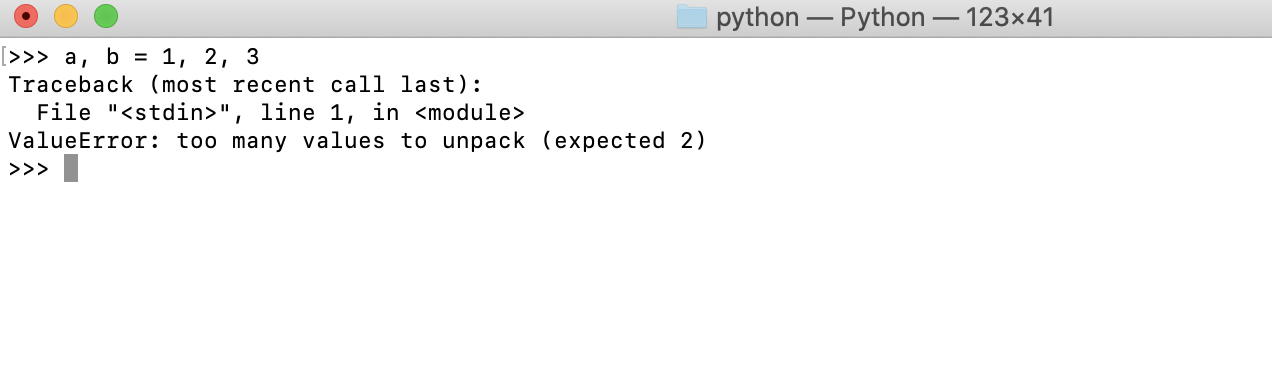## Naming Identifiers and Python Keywords

Python, like other languages, has a couple of rules on naming identifiers such as variable names, class names, function names, module names, and other objects. Some are strictly enforced by the interpreter, while others are simply by convention, and developers are at liberty to ignore them. The rules and conventions are designed to avoid confusion when the interpreter is parsing through code or to make the code more easily readable by human

Step 1 : An identifier can consist of upper and lowercase letters of the alphabet, underscores, unicode identifiers, and digits 0 to 9.

Step 2 : An identifier cannot begin with a digit; for example, 8x is an invalid variable name.

Step 3 : No other characters can be in identifiers. This means spaces or any other symbols. Spaces most notably occur in module names as some operating systems will permit filenames with spaces. This should be avoided.

Step 4 : A Python keyword cannot be used in an identifier name, for example, import, if, for, and lambda.

Step 5 : It should also be noted that Python identifier names are case sensitive. Consider this: